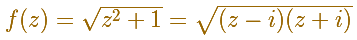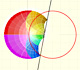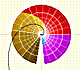We have already seen a multifunction. It is the case of the cubic root that has three values and the point z=0 is called a branch point. Fractional powers are multifunctions.

Now we are considering the two-valued multifunctionWe can draw a path on the left panel and see how this path is transformed on the right panel.

If z travels along a closed loop like in this picture, not encircling any of the two branch points, its image f(z) travels along a closed loop and returns to its original value.However, if z instead travels along a closed loop which goes round only one of the branch points, then f(z) does not return to its original value but instead ends up at a different value of the multifunction.Similarly, if z travels along a closed loop encircling one of the branch points twice, then f(z) returns to its original value again.The same happens if the closed loop goes round both branch points.REFERENCES

Tristan Needham - Visual Complex Analysis. (pag. 96) - Oxford University PressThe usual definition of a function is restrictive. We may broaden the definition of a function to allow f(z) to have many differente values for a single value of z. In this case f is called a many-valued function or a multifunction.Inversion is a plane transformation that transform straight lines and circles in straight lines and circles.Una primera aproximación a estas transformaciones. Representación de dos haces coaxiales de circunferencias ortogonales.The Complex Exponential Function extends the Real Exponential Function to the complex plane.The Complex Cosine Function extends the Real Cosine Function to the complex plane. It is a periodic function that shares several properties with his real ancestor.The Complex Cosine Function maps horizontal lines to confocal ellipses.Examples of complex functions: polynomials, rationals and Moebius Transformations.